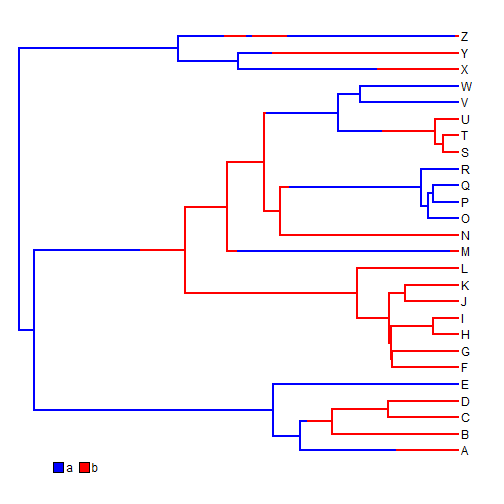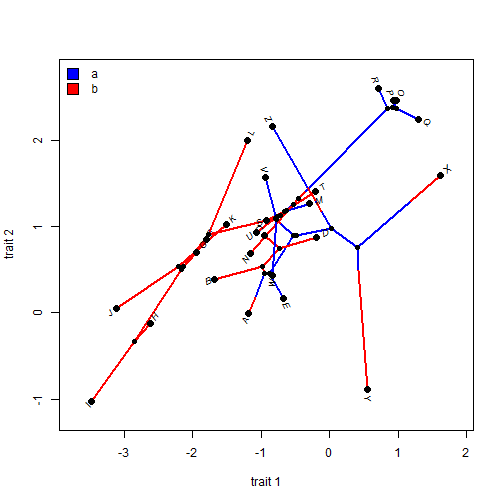## Tuesday, July 28, 2015

### Important bug fix in evol.vcv

A phytools user by the name of Jurriaan de Vos recently identified an important bug in the phytools function `evol.vcv`. `evol.vcv` implements the method of Revell & Collar (2009) for fitting two or more among-trait evolutionary covariance matrices for the Brownian process to different parts of the tree. The problem stems from a bug introduced in which I inadvertently assumed that the order of the input data matrix matched the order of the tip labels in `tree`.

Here is an example of the problem.

First, I'll simulate data with different correlation structure in different parts of the tree:

``````library(phytools)
tree<-pbtree(n=26,tip.label=LETTERS,scale=2)
Q<-matrix(c(-1,1,1,-1),2,2)
rownames(Q)<-colnames(Q)<-letters[1:2]
tree<-sim.history(tree,Q,anc="a")
``````
``````## Done simulation(s).
``````
``````plotSimmap(tree,colors=setNames(c("blue","red"),letters[1:2]),ylim=c(-1,27))
x=0.1*max(nodeHeights(tree)),y=0,vertical=FALSE)
````````````vcv<-list(matrix(c(1,0,0,1),2,2),
matrix(c(2,1.8,1.8,2),2,2))
names(vcv)<-letters[1:2]
vcv
``````
``````## \$a
##      [,1] [,2]
## [1,]    1    0
## [2,]    0    1
##
## \$b
##      [,1] [,2]
## [1,]  2.0  1.8
## [2,]  1.8  2.0
``````
``````X<-sim.corrs(tree,vcv=vcv)
``````
``````phylomorphospace(tree,X,colors=setNames(c("blue","red"),letters[1:2]),
xlab="trait 1",ylab="trait 2")
x=par()\$usr+0.02*(par()\$usr-par()\$usr),
y=par()\$usr-0.04*(par()\$usr-par()\$usr))
``````OK, now let's fit the models:

``````fit1<-evol.vcv(tree,X)
fit1
``````
``````## ML single-matrix model:
##  R[1,1]  R[1,2]  R[2,2]  k   log(L)
## fitted   1.1928  0.7186  0.9892  5   -57.9027
##
## ML multi-matrix model:
##  R[1,1]  R[1,2]  R[2,2]  k   log(L)
## a    0.3866  -0.1434 0.4604  8   -53.3203
## b    1.9253  1.467   1.4903
##
## P-value (based on X^2): 0.0272
##
## R thinks it has found the ML solution.
``````
``````## rows scrambled:
fit2<-evol.vcv(tree,X[sample(rownames(X)),])
fit2
``````
``````## ML single-matrix model:
##  R[1,1]  R[1,2]  R[2,2]  k   log(L)
## fitted   1.1928  0.7186  0.9892  5   -131.4336
##
## ML multi-matrix model:
##  R[1,1]  R[1,2]  R[2,2]  k   log(L)
## a    0.9387  0.6829  1.4885  8   -86.0687
## b    5.4343  2.7888  4.7515
##
## P-value (based on X^2): 0
##
## R thinks it has found the ML solution.
``````

There are obviously multiple weird things going on here. Firstly, the single-matrix model is fit correctly, though the log-likelihood is obviously totally wrong. Secondly, the two-matrix models are completely messed up….

Interestingly, the bug does not affect `evolvcv.lite` which fits the same model - as well as other ones - but only for datasets with just two continuous traits. (Unfortunately, there is no fancy `print` method for `evolvcv.lite`).

``````fit3<-evolvcv.lite(tree,X[sample(rownames(X)),])
fit3\$model1
``````
``````## \$description
##  "common rates, common correlation"
##
## \$R
##           [,1]      [,2]
## [1,] 1.1927796 0.7185548
## [2,] 0.7185548 0.9891478
##
## \$logLik
##  -57.90274
##
## \$convergence
##  0
##
## \$k
##  5
##
## \$AIC
##  125.8055
``````
``````fit3\$model4
``````
``````## \$description
##  "no common structure"
##
## \$R
## \$R\$a
##            [,1]       [,2]
## [1,]  0.3862680 -0.1435202
## [2,] -0.1435202  0.4596485
##
## \$R\$b
##          [,1]     [,2]
## [1,] 1.924823 1.466919
## [2,] 1.466919 1.490986
##
##
## \$logLik
##  -53.32028
##
## \$convergence
##  0
##
## \$k
##  8
##
## \$AIC
##  122.6406
``````

The fix - also pointed out by Jurriaan is extremely simple & merely involved moving one line of code (in which `X`) is vectorized two lines below (to be below where the rows of `X` were already sorted)! I have posted a new version of `evol.vcv` with this fix implemented here and will obviously be in the next update to phytools.

Here's a demo showing that it is fixed:

``````source("http://www.phytools.org/evol.vcv/v0.7/evol.vcv.R")
library(numDeriv)
fit4<-evol.vcv(tree,X[sample(rownames(X)),])
fit4
``````
``````## ML single-matrix model:
##  R[1,1]  R[1,2]  R[2,2]  k   log(L)
## fitted   1.1928  0.7186  0.9892  5   -57.9027
##
## ML multi-matrix model:
##  R[1,1]  R[1,2]  R[2,2]  k   log(L)
## a    0.3866  -0.1434 0.4604  8   -53.3203
## b    1.9253  1.467   1.4903
##
## P-value (based on X^2): 0.0272
##
## R thinks it has found the ML solution.
``````

That's it.